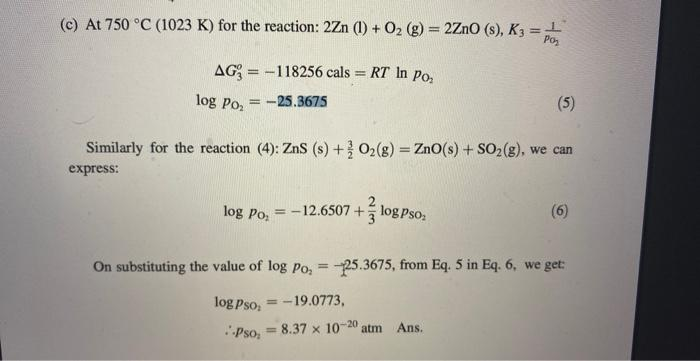Home / Expert Answers / Chemical Engineering / didnt-understand-these-values-corresponding-5-and-6-can-you-calculate-again-by-writing-thanks-acc-pa891

# (Solved): didnt understand these values corresponding 5 and 6 can you calculate again by writing thanks acc ...

? didnt understand these values corresponding 5 and 6 can you calculate again by writing thanks according to my previous question teacher(c) At $$750^{\circ} \mathrm{C}(1023 \mathrm{~K})$$ for the reaction: $$2 \mathrm{Zn}(\mathrm{l})+\mathrm{O}_{2}(\mathrm{~g})=2 \mathrm{ZnO}(\mathrm{s}), K_{3}=\frac{1}{P_{0_{2}}}$$ \begin{aligned} \Delta G_{3}^{o} & =-118256 \mathrm{cals}=R T \ln p_{\mathrm{O}_{2}} \\ \log p_{\mathrm{O}_{2}} & =-25.3675 \end{aligned} Similarly for the reaction (4): $$\mathrm{ZnS}(\mathrm{s})+\frac{3}{2} \mathrm{O}_{2}(\mathrm{~g})=\mathrm{ZnO}(\mathrm{s})+\mathrm{SO}_{2}(\mathrm{~g})$$, we can express: $\log p_{\mathrm{O}_{2}}=-12.6507+\frac{2}{3} \log p_{\mathrm{SO}_{2}}$ On substituting the value of $$\log p_{\mathrm{O}_{2}}=-25.3675$$, from Eq. 5 in Eq. 6, we get: \begin{aligned} \log p_{\mathrm{SO}_{2}} & =-19.0773, \\ \therefore p_{\mathrm{SO}_{2}} & =8.37 \times 10^{-20} \text { atm Ans. } \end{aligned}

We have an Answer from Expert